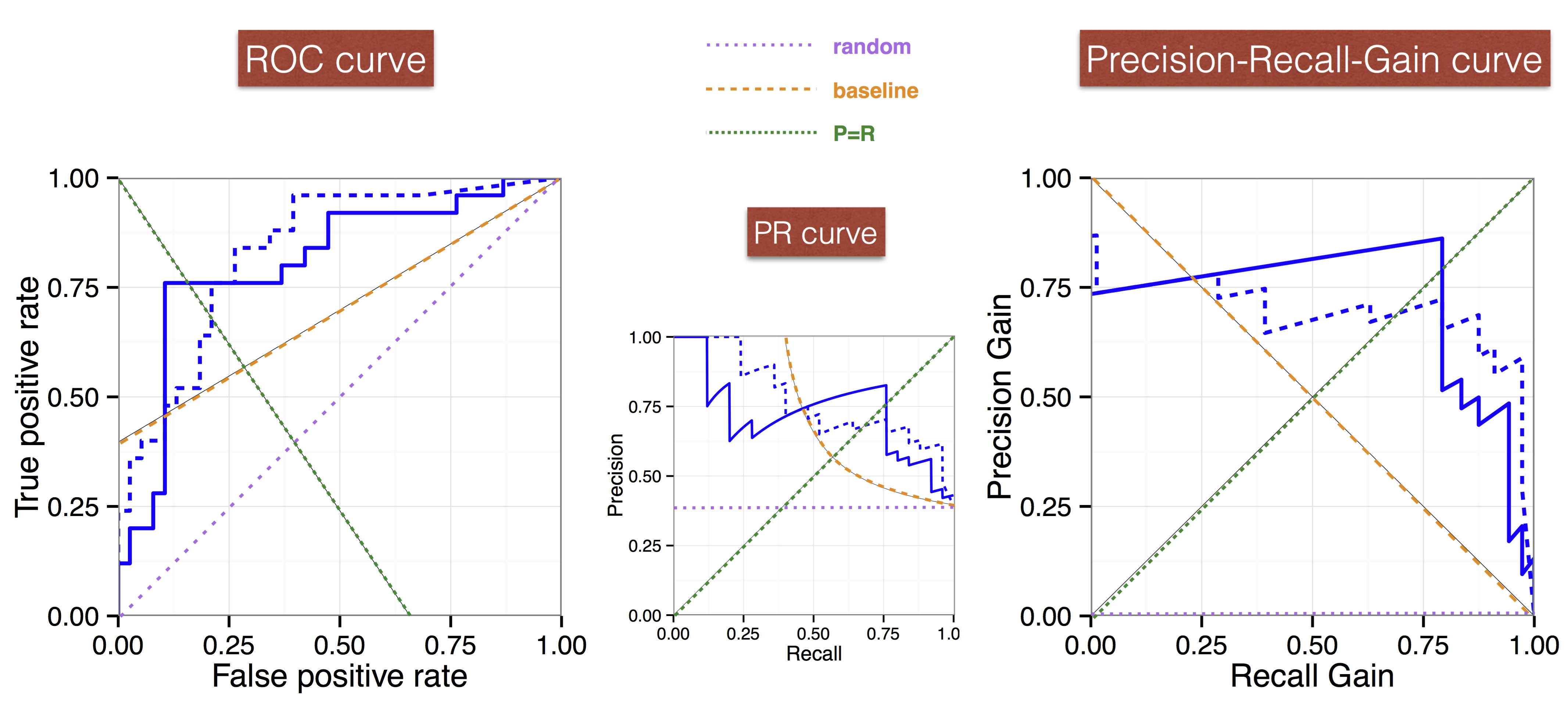# Precision-Recall-Gain Curves: PR Analysis Done Right

## Peter A. Flach and Meelis Kull, Intelligent Systems Laboratory, University of Bristol

Precision-Recall analysis abounds in applications of binary classification where true negatives do not add value and hence should not affect assessment of the classifier's performance. Perhaps inspired by the many advantages of receiver operating characteristic (ROC) curves and the area under such curves for accuracy-based performance assessment, many researchers have taken to report Precision-Recall (PR) curves and associated areas as performance metric. We demonstrate in this paper that this practice is fraught with difficulties, mainly because of incoherent scale assumptions -- e.g., the area under a PR curve takes the arithmetic mean of precision values whereas the $$F_{\beta}$$ score applies the harmonic mean. We show how to fix this by plotting PR curves in a different coordinate system, and demonstrate that the new Precision-Recall-Gain curves inherit all key advantages of ROC curves. In particular, the area under Precision-Recall-Gain curves conveys an expected $$F_1$$ score on a harmonic scale, and the convex hull of a Precision-Recall-Gain curve allows us to calibrate the classifier's scores so as to determine, for each operating point on the convex hull, the interval of $$\beta$$ values for which the point optimises $$F_{\beta}$$. We demonstrate experimentally that the area under traditional PR curves can easily favour models with lower expected $$F_1$$ score than others, and so the use of Precision-Recall-Gain curves will result in better model selection.### Take-home messages:

1. Precision-Recall analysis and F-scores require proper treatment of their harmonic scale — arithmetic averages or linear expectations of F-scores etc are incoherent.
2. Precision-Recall-Gain curves properly linearise the quantities involved and their area is meaningful as an aggregate performance score.
3. These things matter in practice as AUPR can easily favour worse-performing models, unlike AUPRG.
4. Using PRG curves we can identify all $$F_{\beta}$$-optimal thresholds for any $$\beta$$ in a single calibration procedure.